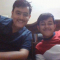Class Central is learner-supported. When you buy through links on our site, we may earn an affiliate commission.

# Introduction to Algorithms

### Overview

Course Features
• Video lectures
• Captions/transcript
• Selected lecture notes
• Assignments: problem sets with solutions
• Assignments: programming with examples
• Exams and solutions
Course Highlights

This course features a complete set of lecture notes and videos. The course textbook was co-written by Prof. Leiserson.

Course Description

This course teaches techniques for the design and analysis of efficient algorithms, emphasizing methods useful in practice. Topics covered include: sorting; search trees, heaps, and hashing; divide-and-conquer; dynamic programming; amortized analysis; graph algorithms; shortest paths; network flow; computational geometry; number-theoretic algorithms; polynomial and matrix calculations; caching; and parallel computing.

This course was also taught as part of the Singapore-MIT Alliance (SMA) programme as course number SMA 5503 (Analysis and Design of Algorithms).

### Syllabus

Lec 1 | MIT 6.046J / 18.410J Introduction to Algorithms (SMA 5503), Fall 2005.
Lec 2 | MIT 6.046J / 18.410J Introduction to Algorithms (SMA 5503), Fall 2005.
Lec 3 | MIT 6.046J / 18.410J Introduction to Algorithms (SMA 5503), Fall 2005.
Lec 4 | MIT 6.046J / 18.410J Introduction to Algorithms (SMA 5503), Fall 2005.
Lec 5 | MIT 6.046J / 18.410J Introduction to Algorithms (SMA 5503), Fall 2005.
Lec 6 | MIT 6.046J / 18.410J Introduction to Algorithms (SMA 5503), Fall 2005.
Lec 7 | MIT 6.046J / 18.410J Introduction to Algorithms (SMA 5503), Fall 2005.
Lec 8 | MIT 6.046J / 18.410J Introduction to Algorithms (SMA 5503), Fall 2005.
Lec 9 | MIT 6.046J / 18.410J Introduction to Algorithms (SMA 5503), Fall 2005.
Lec 10 | MIT 6.046J / 18.410J Introduction to Algorithms (SMA 5503), Fall 2005.
Lec 11 | MIT 6.046J / 18.410J Introduction to Algorithms (SMA 5503), Fall 2005.
Lec 12 | MIT 6.046J / 18.410J Introduction to Algorithms (SMA 5503), Fall 2005.
Lec 13 | MIT 6.046J / 18.410J Introduction to Algorithms (SMA 5503), Fall 2005.
Lec 14 | MIT 6.046J / 18.410J Introduction to Algorithms (SMA 5503), Fall 2005.
Lec 15 | MIT 6.046J / 18.410J Introduction to Algorithms (SMA 5503), Fall 2005.
Lec 16 | MIT 6.046J / 18.410J Introduction to Algorithms (SMA 5503), Fall 2005.
Lec 17 | MIT 6.046J / 18.410J Introduction to Algorithms (SMA 5503), Fall 2005.
Lec 18 | MIT 6.046J / 18.410J Introduction to Algorithms (SMA 5503), Fall 2005.
Lec 19 | MIT 6.046J / 18.410J Introduction to Algorithms (SMA 5503), Fall 2005.
Lec 22 | MIT 6.046J / 18.410J Introduction to Algorithms (SMA 5503), Fall 2005.
Lec 23 | MIT 6.046J / 18.410J Introduction to Algorithms (SMA 5503), Fall 2005.
Lec 24 | MIT 6.046J / 18.410J Introduction to Algorithms (SMA 5503), Fall 2005.
Lec 25 | MIT 6.046J / 18.410J Introduction to Algorithms (SMA 5503), Fall 2005.

### Taught by

Prof. Erik Demaine , Prof. Srini Devadas and Prof. Nancy Lynch

## Reviews

5.0 rating, based on 1 Class Central review

Start your review of Introduction to Algorithms

•DHRUV
IT WAS GREAT . THANK YOU FOR THIS FREE COURSE. This course covers the essential information that every serious programmer needs to know about algorithms and data structures, with emphasis on applications and scientific performance analysis of Java implementations. IT covers elementary data structures, sorting, and searching algorithms AND focuses on graph- and string-processing algorithms.

All the features of this course are available for free. It does not offer a certificate upon completion.

### Never Stop Learning.

Get personalized course recommendations, track subjects and courses with reminders, and more.